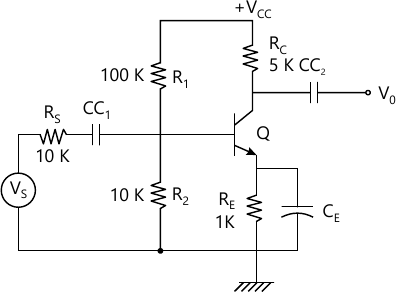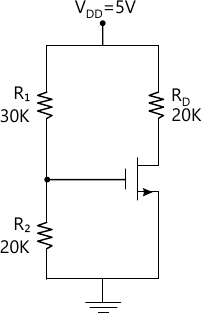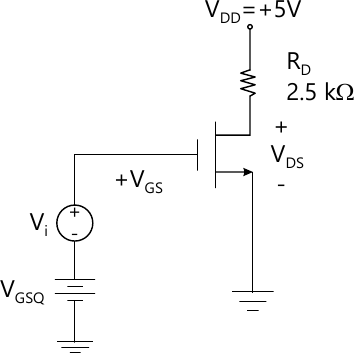MORE IN Electronic Devices & Circuits
SPPU Electronics and Telecom Engineering (Semester 3)
Electronic Devices & Circuits
December 2015
Total marks: --
Total time: --
INSTRUCTIONS
(1) Assume appropriate data and state your reasons
(2) Marks are given to the right of every question
(3) Draw neat diagrams wherever necessary

Solve any one question from Q1 and Q2
1 (a) State and explain three stability factors.
6 M
1 (b) Consider single stage CE amplifier with RS=1 kΩ, R1=50 kΩ, R2=2 kΩ, RC=2 kΩ, RL=2 kΩ, hfe=50, hre=2.5×10-4, hoe=25 μ Amp/V, hie=1.1 kΩ. Calculate: Ai, Ri and R0.
6 M

2 (a) Explain diode compensation technique against ICO.
6 M
2 (b) Calculate AVS, Ais & R0 for the transistor amplifier shown in Fig. (1) having h-parameters hie=1.1 k hfe=50, hre=2.5×10-4, hoe=1/40k.6 M

Solve any one question from Q3 and Q4
3 (a) Draw and explain low frequency response of single stage RC coupled CE amplifier.
6 M
3 (b) Determine the frequency of Oscillation when RC phase shift oscillator has R=10 k, C=0.01 μf and RC=2.2k. Also find the minimum current gain needed for this purpose.
6 M

4 (a) The following measurement were taken while testing an amplifier using square wave input waveform:
i) for frequency of 5 kHz, tr=20 μsec.
ii) for frequency of 100 Hz, there is a sag/tilt of 1 volt in 2.5 volts.
Amplitude as observed on CRO. Determine the bandwidth of the amplifier under test.
6 M
4 (b) Draw and explain Hartley oscillator.
6 M

Solve any one question from Q5 and Q6
5 (a) Draw and explain vertically oriental structure of n-p-n power BJT.
6 M
5 (b) Class A power amplifier has zero signal collector current of 100 mA. If the collector supply voltage is 5 V, determine:
i) Maximum AC power output
ii) Power rating of transistor
iii) Maximum collector circuit efficiency.
7 M

6 (a) Draw and explain class B-push pull power amplifier. State its merits and demerits.
7 M
6 (b) A power amplifier supplies 3 watt to a load of 6 kΩ. The zero signal DC collector current is 55 mA and the collector current with signal is 60 mA. How much is the percentage second harmonic distortion?
6 M

Solve any one question from Q7 and Q8
7 (a) Explain the following non-ideal current voltage characteristics of MOSFET:
i) Finite output resistance
ii) Body effect
iii) Subthreshold conduction.
6 M
7 (b) Calculate the drain current and drain to source voltage of common source circuit shown in Fig. 2. Given: VTN=1 V, Kn=0.1 mA/V2.7 M

8 (a) Draw and explain constant current source biasing circuit for EMOSFET.
6 M
8 (b) For the circuit shown in Fig 3. determine the small signal voltage gain. Assume parameters VGSQ=2.12 V, VDD=5 V, RD=2.5 kΩ, VTN=1 V, Kn=0.8 mA/V2, λ=0.02 V-1. Assume the transistor is biased in the saturation region.7 M

More question papers from Electronic Devices & Circuits